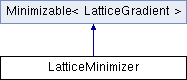JDFTx  1.4.2LatticeMinimizer Class Reference

Lattice minimizer. More...

#include <LatticeMinimizer.h>

Inheritance diagram for LatticeMinimizer:## Public Member Functions

LatticeMinimizer (Everything &)

void step (const LatticeGradient &dir, double alpha)
Move the state in parameter space along direction dir with scale alpha.

double compute (LatticeGradient *grad, LatticeGradient *Kgrad)
Returns the objective function at the current state and store the gradient in grad and preconditioned gradient in Kgrad, if non-null.

bool report (int iter)

void constrain (LatticeGradient &)
Constrain search directions to the space of free directions for minimize.

double safeStepSize (const LatticeGradient &dir) const
Override to return maximum safe step size along a given direction. Steps can be arbitrarily large by default.

double sync (double x) const
All processes minimize together; make sure scalars are in sync to round-off error.

void calculateStress ()
calculate current stress (in Eh/a0^3 units) and store to IonInfo::stress

double minimize (const MinimizeParams &params)
minor addition to Minimizable::minimize to invoke charge analysis at final positionsPublic Member Functions inherited from Minimizable< LatticeGradient >
double minimize (const MinimizeParams &params)
Minimize this objective function with algorithm controlled by params and return the minimized value.

void fdTest (const MinimizeParams &params)

## Friends

class IonDynamics

## Detailed Description

Lattice minimizer.

## Member Function Documentation

 bool LatticeMinimizer::report ( int iter )
virtual

Override for optional processing/reporting after each/every few iterations It should return whether the state was modified

Reimplemented from Minimizable< LatticeGradient >.

The documentation for this class was generated from the following file: